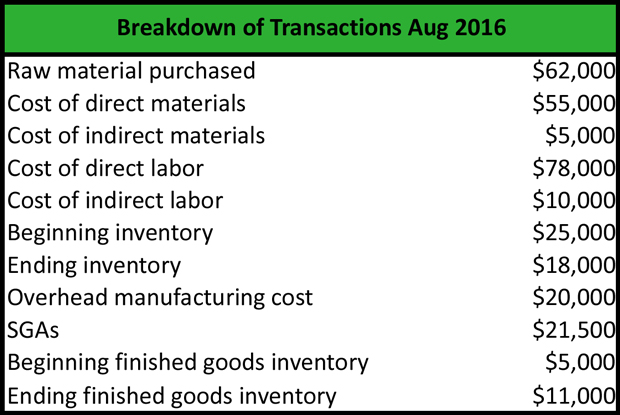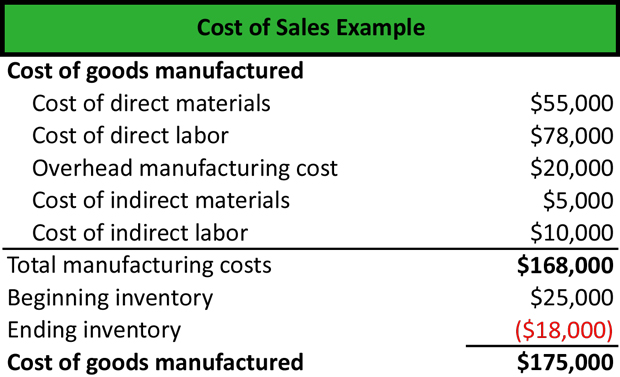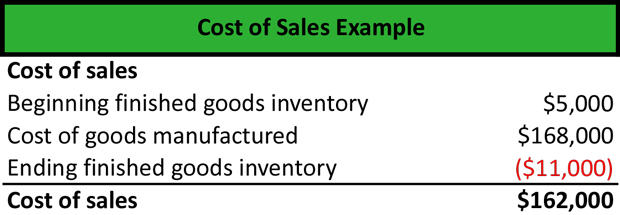# What is Cost of Sales?

Definition: The cost of sales, also known as the cost of goods sold (COGS), represents the direct costs related to the manufacturing or purchasing of a good that is sold to a customer. Companies use this measurement to calculate their gross margin.

## What Does Cost of Sales Mean?

What is the definition of cost of sales? The cost of sales formula can be calculated two different ways. You can adjust the cost of the goods purchased or manufactured by the change in inventory during a given period. You can also add the cost of goods purchased or manufactured to the inventory at the beginning of the period and subtract the inventory of goods at the end of the period.

The main components we need to calculate the cost of sales equation is the beginning inventory, the cost of direct materials, the cost of direct labor, the overhead costs, the ending inventory, and the cost of acquiring or manufacturing new products.

Let’s look at an example.

## Example

Company ABC is a manufacturing company that manufactures heating systems. Mary, the company’s accountant, is asked to calculate the cost of sales based on the company’s transactions in August. Mary creates a breakdown of transactions with the following information:First, Mary calculates the total manufacturing costs by summing up the cost of direct materials: the cost of direct labor, the overhead manufacturing cost, the cost of indirect materials, and the cost of indirect labor. The total manufacturing costs are \$168,000.

Second, Mary adds the beginning inventory and subtracts the ending inventory to calculate the cost of goods manufactured, which is \$175,000.Third, Mary calculates the cost of sales by adding the cost of goods manufactured to the beginning inventory of finished goods and subtracting the ending inventory of finished goods. The cost of sales for August is \$162,000.To calculate the COS, Mary does not take into consideration the SGAs (selling, general and administrative expenses) as well as the raw materials purchased. The cost of goods sold includes the cost of goods manufactured of purchased plus the inventory at the beginning of the period minus the inventory at the end of the period.

## Summary Definition

Define Cost of Sales: COS consists of the expenses associated with purchasing or making a product that has been sold to a customer.